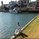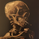900 views
The Chauvenet criterion is a well-known criterion of selection and rejection of the data used by the Physics. It establishes that in an experiment is well to discard the data whose distance from the average is greater than a certain number of the delta.
In the stock market if prices move away from the average with a volatility too high are suspect. This principle is embodied in the Chauvenet floor with the definition of two asymptotes and two data areas rejection.
The Chauvenet Radius is the quadratic sum of the delta (distance from average) and sigmoid ( volatility ) and is therefore an obvious market stability index. In fact the moments when price strongly moves away from the average with high volatility coincide with the moments of high instability of the market.

It can be considered an evolution of John Bollinger method introduced during the '80.

```//@version=2
len=input(defval=20,minval=1)
price=close
avg=sma(price,len)
x=price-avg
y=stdev(price,len)
histpos=hist1<0?0:hist1
histneg=hist2<0?0:hist2
plot(ema,color=red,transp=80)
plot(histpos,style=columns,color=green)
plot(histneg,style=columns,color=maroon)```